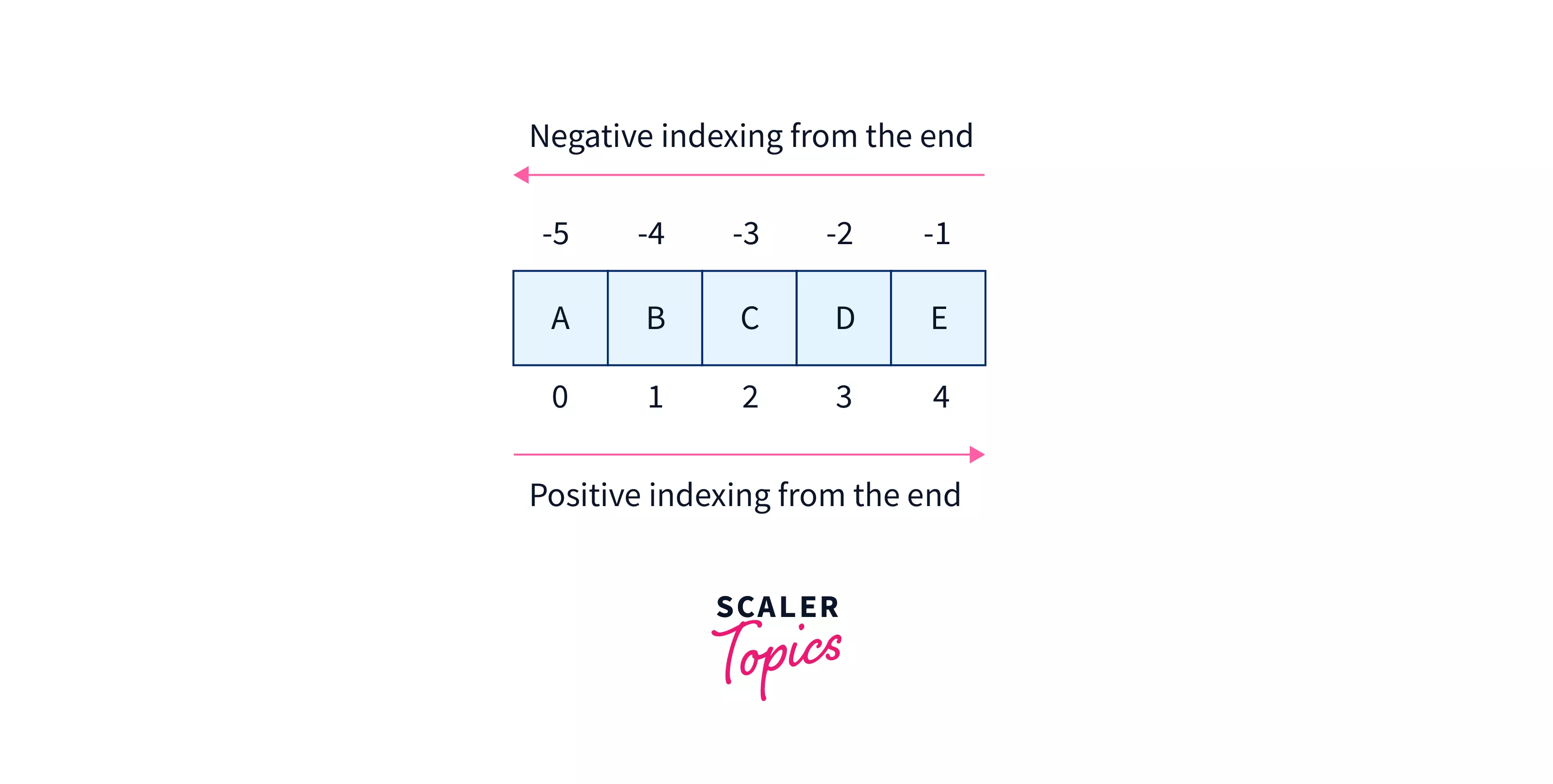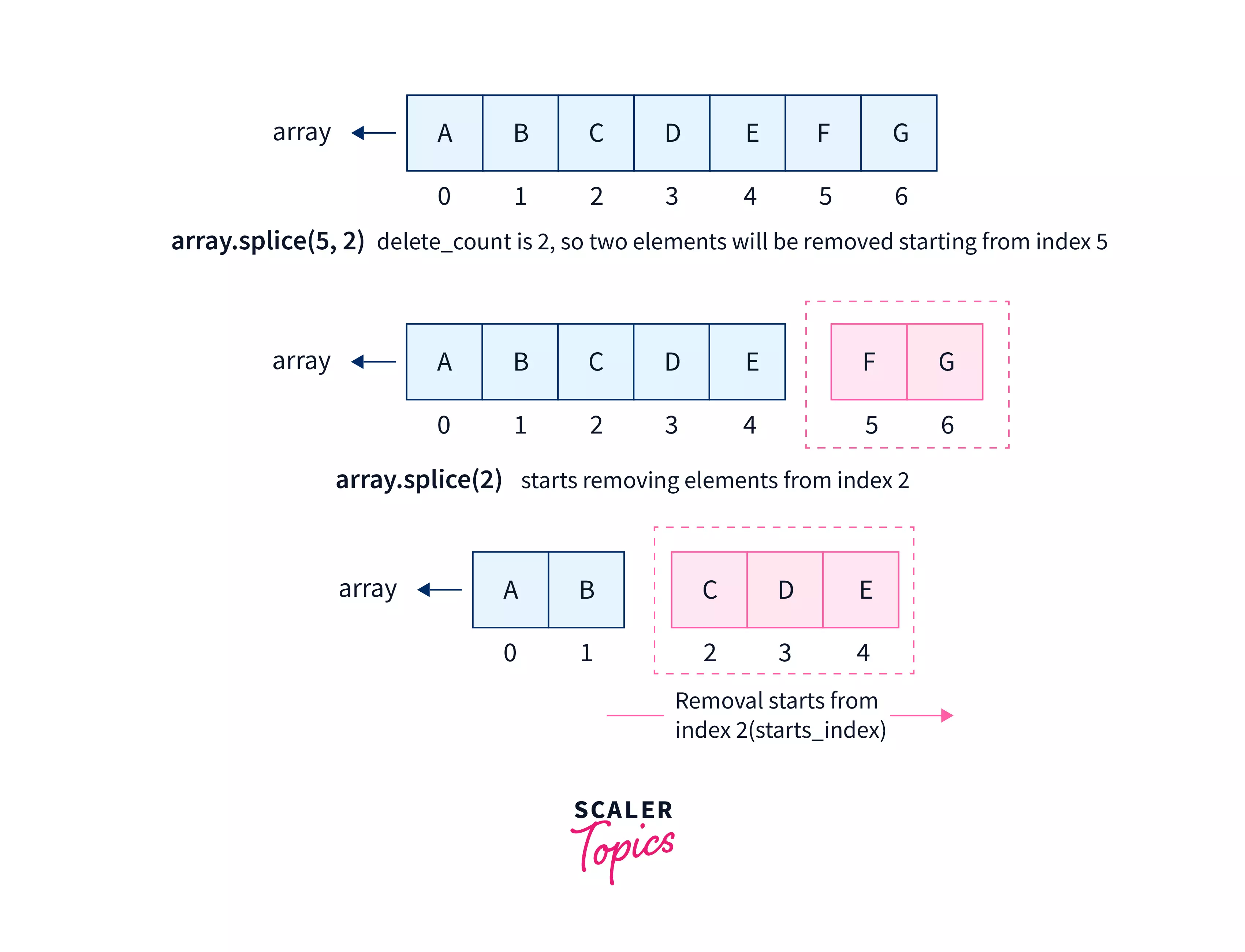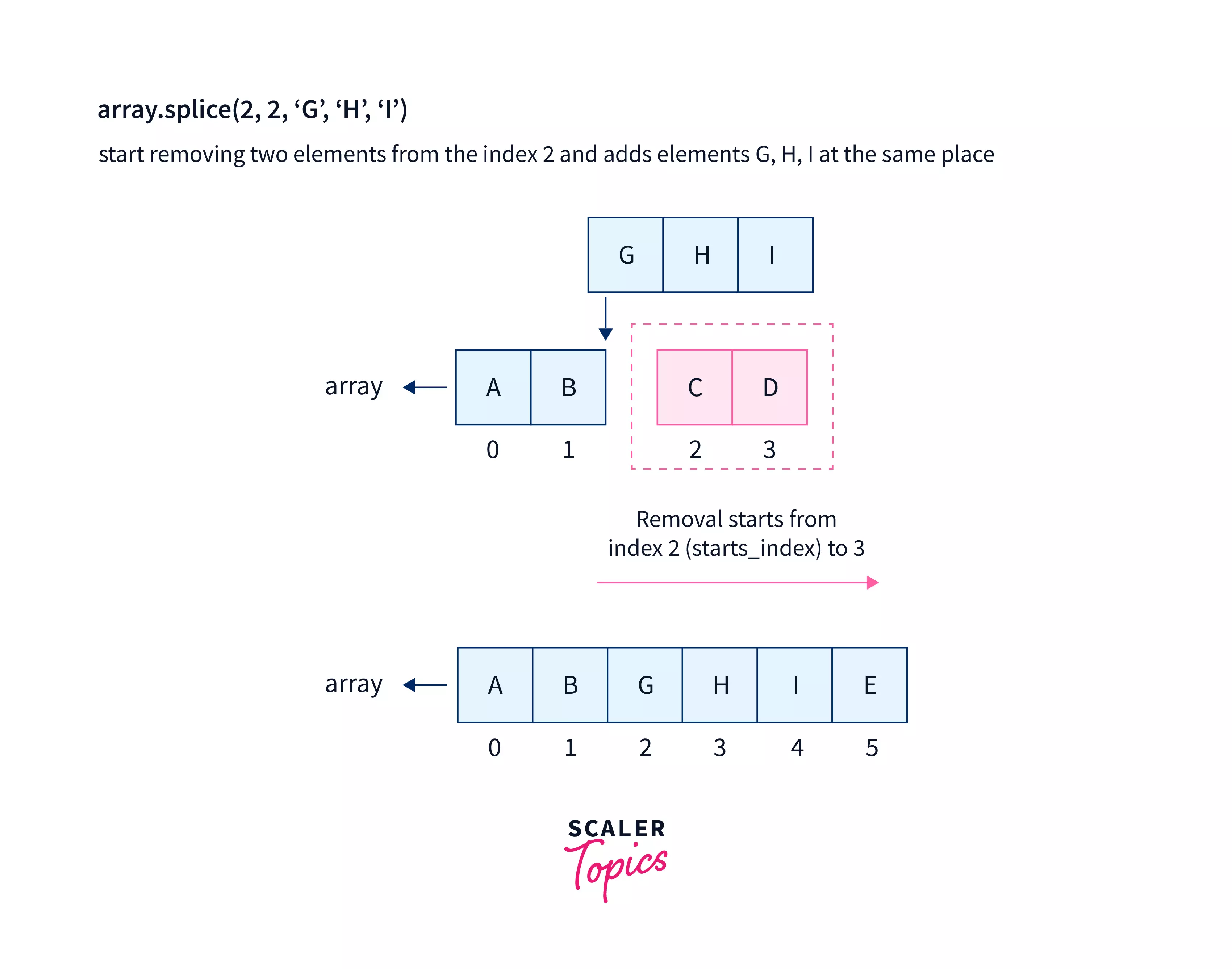# Splice Method in JavaScript

Challenge Inside! : Find out where you stand! Try quiz, solve problems & win rewards!
Learn via video courseJavaScript Course With Certification: Unlocking the Power of JavaScript
By Mrinal BhattacharyaFree
4.8Enrolled: 1000JavaScript Course With Certification: Unlocking the Power of JavaScript
Mrinal BhattacharyaFree
4.8
Enrolled: 1000

## Overview

The splice() method of the Array class in JavaScript modifies the original array by changing its content. It works by removing the existing elements and adding new ones, which might change the array's length.

## What is Array splice() in JavaScript?

The splice in JavaScript is used to modify the contents of the array. It removes the existing elements and/or adds new elements, thereby changing the length of the original array.

As per the syntax, splice method in JavaScript accepts three parameters, of which start_index is an important parameter. On the contrary, the parameters delete_count and the specified elements are optional.

Introducing the parameter delete_count will remove the mentioned number of elements starting from the given index.

Also, if the elements to be added are mentioned, they will be added to the array right from the starting index position.

### Example

Let us take an example of an array containing integers and visualize how the splice() method would work on it.

Output:

## Syntax of Array splice() in Javascript

The syntax of the splice() method is:

Alternatives:

## Parameters of Array splice() in Javascript

The splice() method accepts three parameters:

• start_index (required): start_index is an integer that shows the index from where the modification would take place. start_index is a mandatory parameter that begins at the0th index from the beginning of the array when it is specified to be non-negative

When it is negative, the counting would start from the end of the array starting with -1.• delete_count (optional): delete_count is an integer that denotes the number of elements to be removed from the array. The removal begins at the start_index and goes up to the number of elements as specified by the parameter, delete_count. If not needed, the value delete_count should be 0 because if delete_count is ignored, all the elements starting from start_index are removed.• element1, element2,..., elementN (optional): Since Arrays in JavaScript are objects, it can accept elements of different data types. It denotes the elements that will be added right from the start_index position. If it has not been specified, then the splice method only removes the existing elements from the array and doesn't add any new elements.## Return Value of Array splice() in Javascript

Return Type: Array

The splice in JavaScript returns an array containing all the elements that have been removed from the original array.

Note: If no element has been removed, an empty array will be returned.

## Examples for Understanding

### 1. Using splice() Method

In the coming example, we'll remove two elements from index 3 (start_index) and add two elements. The starting point for the new elements to be added will be the start_index itself.

Output:

### 2. Using splice() for Different delete_count Values

If delete_count is less than or equal to 0, then none of the elements will be removed.

Output:

In a scenario where delete_count is omitted or made greater than the remaining elements of the array from the start_index to the end, then all the elements will be deleted from start_index to the end, i.e.,

Output:

### 3. Using splice() for Different Start Values

Case 1: When start_index is greater than or equal to the length of the array If the start_index is greater than or equal to the length of the array, no element will be removed, and the new elements (if passed as arguments) will be added to the end of the array.

Output:

Case 2: When start_index is less than 0. If the start_index is negative, then the elements would be counted from the end of the array. So, the last element's index would be -1 from the end.

The indexing from the start will be as follows:

Output:

Case 3: When the sum of start_index and length of the array is less than 0. If start_index + array.length < 0, which means it's beyond the scope of backward indexing as well. So, the indexing will start from 0 itself.

For example, let start_index be -9 and array.length is only 6, so their addition still yields a negative value (-3). So, the indexing will start from index 0.

Output:

In the above example, you can observe that the delete_count was 1, so the element at index 0 was removed, and new elements X, Y, Z were added.

## Browser compatibility

The splice method in javascript is supported by all the browsers such as Chrome, Edge, Safari, Opera etc.

## Conclusion

• The splice in JavaScript removes the existing elements and adds newer ones to the array. While the parameters delete_count and elements to be added are optional, the start_index is a necessary parameter.
• If only the start_index is passed as a parameter, the splice() method will start removing all the elements from the start_index to the end of the array. The same result occurs in the case when delete_count >= (array.length - start_index).
• If delete_count<=0, then no elements will be removed from the array.
• If start_index >= array.length, none of the elements will be removed, and new elements (if passed as arguments) will be added to the end of the array.
• If start_index<0, the indexing starts from the end of the array with the last element having the index -1.
• If start_index + array.length < 0, the indexing starts from index 0.